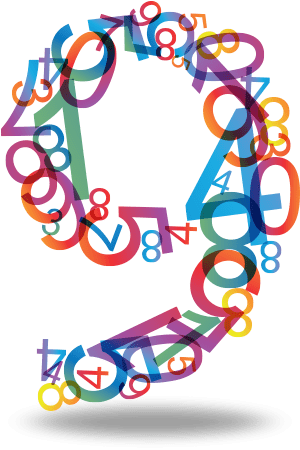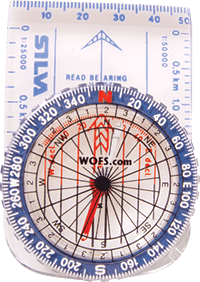# Why the Number 9 Is the Most Supreme of All Numbers?The Master Number 9 is an important number signifying wholeness and completion exactly as Lillian has taught, and while attending the recent MPC in feng shui in Malaysia, I also discovered that all the angles and “degrees” of the compass, the flight of the “stars” in the Lo Shu chart with 5 in the centre that is the basis of all flying star charts expressed as numbers in the three by three grid… all added up to 9!

Not surprising as 9 is the highest digit before we go back to 1.

The Lo Shu square in Feng Shui has nine numbers placed in an arrangement with each number in each grid so that any three numbers in a straight line – whether horizontally, vertically or diagonally add up to 15, which as Lillian explained is the number of days it takes the moon to reach fullness. It doesn’t matter which way you add the numbers they add to 15. The Lo Shu square is the magic square of three. It is also the square of the planet Saturn, so 3X3 =9! Feng Shui definitely has a correlation to astrology, which I see as the basis of all systems, and this can be proven mathematically.

Note that when you add up all the number 1 to 9, i.e. 1+2+3+4+5+6+7+8+9 they add up to 45 degrees, representing each major direction on the compass, and here we see that once again 4+5 also adds up to 9.

### The 9 hidden in the COMPASS and the Feng Shui formulasThe number 9 transcends the feng shui compass, and all the formulas of feng shui thus have the 9 hidden in each of the direction houses. Take the Eight Mansions formula for instance. According to this formula, there are eight directions major direction categories and these are 45 degrees each. Eight times forty-five equals three hundred and sixty degrees which is one circle or a complete cycle. Note that all the important 45 degree angles add up to nine. One eighth = 45degrees, (4+5=9),one quarter =90 degrees (9+0=9),three eights =135 degrees (1+3+5=9),one half =180 degrees (1+8+0=9), five eights = 225 degrees (2+2+5=9),three quarters = 270 degrees (2+7+0=9), seven eights =315 degrees (3+1+5=9) and the last comes back to 360 degrees( 3+6+0 =9). So all the important angles add up to 9! This suggests that 9 is the most important number in feng shui formulas that use the compass.

### Connection to STOCK MARKET

The Chinese agricultural (HSIA or solar) calendar starts on the 4th February each year; the 4th February on the astrological calendar is 315 degrees and this too adds up to the number nine. The astrological year starts on the 21st March each year and if you add 45 degrees to the starting point of zero degrees Aries you end up with the following eight dates which are important turning points in the stock markets, commodities and currencies.

NOTE THESE DATES – they are turning points for the financial markets. We have underlined the dates that are coming soon.

The 6th May (45 degrees), 22nd June (90 degrees), 8th August (135 degrees), 22nd September (180 degrees), 7th November (225 degrees), 22nd December (270 degrees), 4th February (315 degrees) and back to the beginning again completing the circle or cycle.